# Electrical Engineering - EE 2013 GATE Paper (Practice Test)

## 65 Questions MCQ Test Mock Test Series for Electrical Engineering (EE) GATE | Electrical Engineering - EE 2013 GATE Paper (Practice Test)

Description
This mock test of Electrical Engineering - EE 2013 GATE Paper (Practice Test) for GATE helps you for every GATE entrance exam. This contains 65 Multiple Choice Questions for GATE Electrical Engineering - EE 2013 GATE Paper (Practice Test) (mcq) to study with solutions a complete question bank. The solved questions answers in this Electrical Engineering - EE 2013 GATE Paper (Practice Test) quiz give you a good mix of easy questions and tough questions. GATE students definitely take this Electrical Engineering - EE 2013 GATE Paper (Practice Test) exercise for a better result in the exam. You can find other Electrical Engineering - EE 2013 GATE Paper (Practice Test) extra questions, long questions & short questions for GATE on EduRev as well by searching above.
QUESTION: 1

Solution:
QUESTION: 2

Solution:
QUESTION: 3

### In the summer of 2012, in New Delhi, the mean temperature of Monday to Wednesday was 41ºC and of Tuesday to Thursday was 43ºC. If the temperature on Thursday was 15% higher than that of Monday, then the temperature in ºC on Thursday was

Solution:

Let the temperature of Monday be TM
Sum of temperatures of Tuesday and Wednesday = T and
Temperature of Thursday =TTh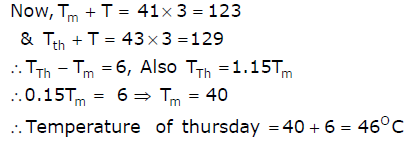QUESTION: 4

Complete the sentence:

Dare ____________ mistakes.

Solution:
QUESTION: 5

They were requested not to quarrel with others.

Which one of the following options is the closest in meaning to the word quarrel?

Solution:
QUESTION: 6

Q. No. 6 – 10 Carry Two Marks Each

A car travels 8 km in the first quarter of an hour, 6 km in the second quarter and 16km in the third quarter. The average speed of the car in km per hour over the entire journey is

Solution:

Average speed = Total distance/Total time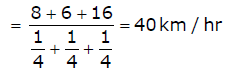QUESTION: 7

If the current changes from 3A to 5A in 2s and the emf is 10V. Calculate the inductance.

Solution:
QUESTION: 8

Statement: There were different streams of freedom movements in colonial India carried out by the moderates, liberals, radicals, socialists, and so on. Which one of the following is the best inference from the above statement?

Solution:
QUESTION: 9

The set of values of p for which the roots of the equation 3x2 + 2x + p (p − 1) = 0 are of opposite sign is

Solution:
QUESTION: 10

What is the chance that a leap year, selected at random, will contain 53 Sundays?

Solution:

There are 52 complete weeks in a calendar year  852 × 7 = 364 days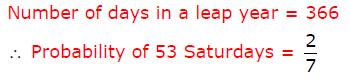QUESTION: 11

Q. No. 11 – 35 Carry One Mark Each

Given a vector field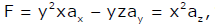the line integral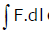evaluated along a segment on the x-axis from x=1 to x=2 is

Solution:
QUESTION: 12

The equation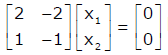has

Solution:
QUESTION: 13

Square roots of −i, where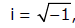are

Solution:
QUESTION: 14

Three moving iron type voltmeters are connected as shown below. Voltmeter readings are V, V1 and V2 as indicated. The correct relation among the voltmeter readings is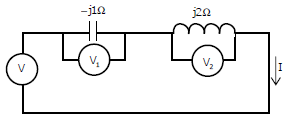Solution:
QUESTION: 15

Leakage flux in an induction motor is

Solution:
QUESTION: 16

The angle δ in the swing equation of a synchronous generator is the

Solution:
QUESTION: 17

Consider a delta connection of resistors and its equivalent star connection as  shown below.  If all elements of the delta connection are scaled by a factor k, k>0, the elements of the corresponding star equivalent will be scaled by a factor of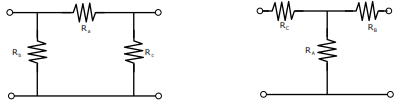Solution:
QUESTION: 18

A band-limited signal with a maximum frequency of 5 kHz is to be sampled. According to the sampling theorem, the sampling frequency in kHz which is not valid is

Solution:
QUESTION: 19

For a periodic signal v(t)=30sin 100t + 10cos 300t + 6 sin(500t+π/4), the fundamental frequency in radians/s is

Solution:
QUESTION: 20

A bulb in a staircase has two switches, one switch being at the ground floor and the other one at the first floor. The bulb can be turned ON and also can be turned OFF by any one of the switches irrespective of the state of the other switch. The logic of switching of the bulb resembles

Solution:
QUESTION: 21

The Bode plot of a transfer function G(s) is shown in the figure below.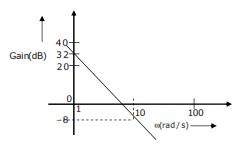The gain (20log|G(s)|) is 32 dB and -8 dB at 1 radians/s and 10 radians/s respectively. The phase is negative for all ω. Then G(s) is

Solution:
QUESTION: 22

In the feedback network shown below, if the feedback factor k is increased, then the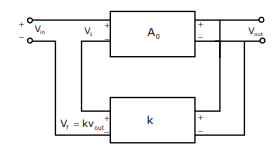Solution:
QUESTION: 23

The input impedance of the permanent magnet moving coil (PMMC) voltmeter is infinite.  Assuming that the diode shown in the figure below is ideal, the reading of the voltmeter in Volts is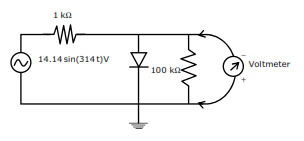Solution:
QUESTION: 24

The curl of the gradient of the scalar field defined by V = 2x2y+3y2z+4z2x is=

Solution:
QUESTION: 25

A continuous random variable X has a probability density function f(x) = e-x , 0 < x < ∞, Then P{X > 1 } is

Solution:
QUESTION: 26

The flux density at a point in space is given by B = 4xa+ 2kyay + 8az Wb / m2  The value of constant k must be equal to

Solution:
QUESTION: 27

A single-phase transformer has no-load loss of 64 W, as obtained from an open-circuit test.  When a short-circuit test is performed on it with 90% of the rated currents flowing in its both LV and HV windings, he measured loss is 81 W.  The transformer has maximum efficiency when operated at

Solution:
QUESTION: 28

A single-phase load is supplied by a single-phase voltage source. If the current flowing from the load to the source is 10∠ - 150° A and if the voltage at the load terminals is 100∠60°V , then the

Solution:
QUESTION: 29

A source Vs(t) = V cos100π t has an internal impedance of( 4+ j3)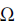. If a purely resistive load connected to this source has to extract the maximum power out of the source, its value inshould be

Solution:
QUESTION: 30

Two systems with impulse responses  h1 (t) and h2 (t) are connected in cascade. Then the overall impulse response of the cascaded system is given by

Solution:
QUESTION: 31

Which one of the following statements is NOT TRUE for a continuous time causal and stable LTI system?

Solution:
QUESTION: 32

The impulse response of a system is h(t) = tu(t). For an input u(t 1),the output is

Solution:
QUESTION: 33

Assuming zero initial condition, the response y(t) of the system given below to a unit step input u(t) is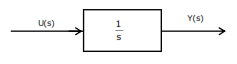Solution:
QUESTION: 34

The transfer function V2(s) / V1(s) of the circuit shown below is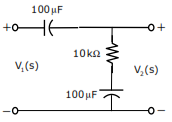Solution:
QUESTION: 35

In the circuit shown below what is the output voltage (Vout ) in Volts if a silicon transistor Q and an ideal op-amp are used?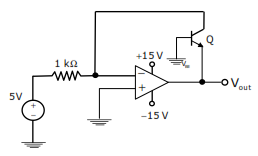Solution:
QUESTION: 36

Q. No. 36 – 65 Carry Two Marks Each

When the Newton-Raphson method is applied to solve the equation f(x) = x3 + 2x -1 = 0 , the solution at the end of the first iteration with the initial guess value as x= 1.2  is

Solution:
QUESTION: 37

A function y= 5x2 +10x is defined over an open interval x = (1,2). Atleast at one point in this interval, dy/dx is exactly

Solution:
QUESTION: 38

A 4-pole induction motor, supplied by a slightly unbalanced three-phase 50Hz source, is rotating at 1440 rpm. The electrical frequency in Hz of the induced negative sequence current in the rotor is

Solution:
QUESTION: 39

Thyristor T in the figure below is initially off and is triggered with a single pulse of width 10 sµ . It is given that L = (100/π)µH and C = (100/π)µF. Assuming latching and holding currents of the thyristor are both zero and the initial charge on C is zero, T conducts for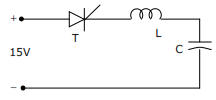Solution:
QUESTION: 40

The following arrangement consists of an ideal transformer and an attenuator which attenuates by a factor of 0.8. An ac voltage Vwx1 = 100V is applied across WX to get an open circuit voltage  across YZ. Next, an ac voltage Vyz2 = 100V is applied across YZ to get an open circuit voltage Vwx2 across WX. Then,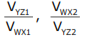are respectively,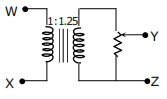Solution:
QUESTION: 41

Two magnetically uncoupled inductive coils have Q factors q1 and q2 at the chosen operating frequency. Their respective resistances are R1 and R1. When connected in series, their effective Q factor at the same operating frequency is

Solution:
QUESTION: 42

The impulse response of a continuous time system is given by h(t)=  δ(t-1) + δ (t-3).  The value of the step response at t = 2 is

Solution:
QUESTION: 43

The signal flow graph for a system is given below. The Transfer function, Y(s)/U(s) for the system is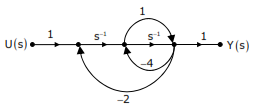Solution:
QUESTION: 44

In the circuit shown below the op-amps are ideal. Then Vout  in Volts is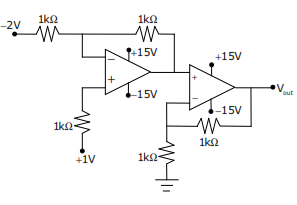Solution:
QUESTION: 45

In the circuit shown below, Qhas negligible collector-to-emitter saturation voltage and the diode drops negligible voltage across it under forward bias. If Vcc is +5V, X and Y are digital signals with 0V as logic 0 and Voc as logic 1, then the Boolean expression for Z is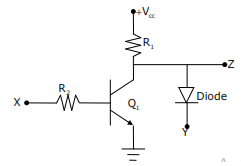Solution:
QUESTION: 46

The clock frequency applied to the digital circuit shown in the figure below is 1kHz. If the initial state of the output of the flip-flop is 0, then the frequency of the output waveform Q in kHz is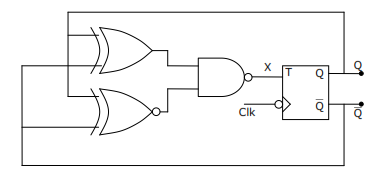Solution:
QUESTION: 47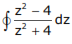evaluated anticlockwise around the circle z - i =2, where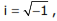is

Solution:
QUESTION: 48

A Matrix has eigenvalues -1 and -2. The corresponding eigenvectors are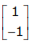and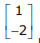respectively. The matrix is

Solution:
QUESTION: 49

A dielectric slab with 500mm x 500mm cross-section is 0.4m long. The slab is subjected to a uniform electric field of E = 6ax + 8ay  kV / mm. The relative permittivity of the dielectric material is equal to 2. The value of constant ε0  is 8.85x10-12 F/m. The energy stored in the dielectric in Joules is

Solution:
QUESTION: 50

For a power system network with n nodes, Z33 of its bus impedance matrix is j0.5 per unit. The voltage at node 3 is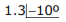per unit. If a capacitor having reactance of -j3.5 per unit is now added to the network between node 3 and the reference node, the current drawn by the capacitor per unit is

Solution:
QUESTION: 51

The separately excited dc motor in the figure below has a rated armature current of 20A and a rated armature voltage of 150V. An ideal chopper switching at 5 kHz is used to control the armature voltage. If La = 0.1mH,  Ra = 1, neglecting armature reaction, the duty ratio of the chopper to obtain 50% of the rated a a torque at the rated speed and the rated field current is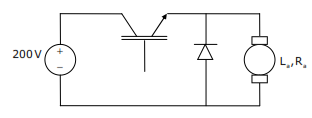Solution:
QUESTION: 52

A voltage 1000 sin ωt Volts is applied across YZ. Assuming ideal diodes, the voltage measured across WX in Volts is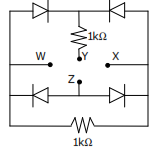Solution:
QUESTION: 53

Three capacitors C1 ,  C2 , and C3  whose values are 10 µF,  5 µF and 2 µF respectively, have breakdown voltages of 10V, 5V and 2V respectively. For the interconnection shown, the maximum safe voltage in Volts that can be applied across the combination and the corresponding total charge in µC stored in the effective capacitance across the terminals are respectively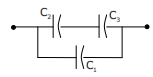Solution:
QUESTION: 54

In the circuit shown below, if the source voltage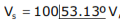then the Thevenin’s equivalent voltage in volts as seen by the load resistance RL is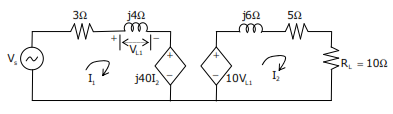Solution:
QUESTION: 55

The open loop transfer function of a dc motor is given as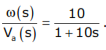When connected in feedback as shown below, the approximate value of Kthat will reduce the time constant of the closed loop system by one hundred times as compared to that of the open loop system is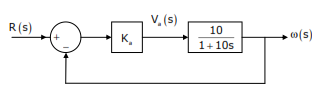Solution:
QUESTION: 56

In the circuit shown below, the knee current of the ideal Zener diode is 10mA. To maintain 5V across RL, the minimum value of RL inand the minimum power rating of the Zener diode in mW respectively are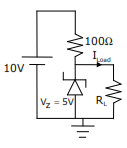Solution:
QUESTION: 57

A strain gauge forms one arm of the bridge shown in the figure below and has a  nominal resistance without any load as Rs = 300. Other bridge resistances are R1 = R2 = R3 = 300. The maximum permissible current through the strain gauge is 20mA. During certain measurement when the bridge is excited by maximum permissible voltage and the strain gauge resistance is increased by 1% over the nominal value, the output voltage V0  in mV is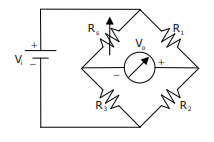Solution:
QUESTION: 58

Common Data Question: 58 & 59

The state variable formulation of a system is given as: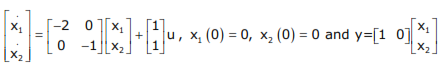The response y(t) to the unit step input is

Solution:
QUESTION: 59

The state variable formulation of a system is given as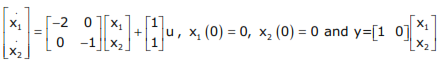The system is

Solution:
QUESTION: 60

Common Data Questions: 60 & 61

In the figure shown below, the chopper feeds a resistive load from a battery source. MOSFET Q is switched at 250 kHz, with a duty ratio of 0.4. All elements of the circuit are assumed to be ideal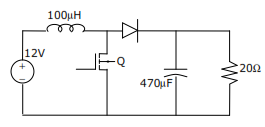The Peak to Peak source current ripple in amps is

Solution:
QUESTION: 61

In the figure shown below, the chopper feeds a resistive load from a battery source. MOSFET Q is switched at 250 kHz, with a duty ratio of 0.4. All elements of the circuit are assumed to be ideal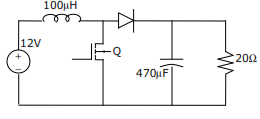The average source current in Amps in steady-state is

Solution:
QUESTION: 62

In the following network, the voltage magnitudes at all buses are equal to 1 pu, the voltage phase angles are very small, and the line resistances are negligible. All the line reactances are equal to j1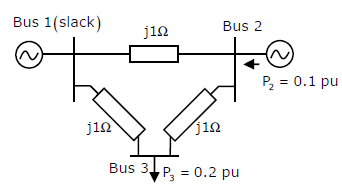The voltage phase angles in rad at buses 2 and 3 are

Solution:
QUESTION: 63

In the following network, the voltage magnitudes at all buses are equal to 1 pu, the voltage phase angles are very small, and the line resistances are negligible. All the line reactances are equal to j1If the base impedance and the line-to line base voltage are 100 ohms and 100kV respectively, then the real power in MW delivered by the generator connected at the slack bus is

Solution:
QUESTION: 64

The Voltage Source Inverter (VSI) shown in the figure below is switched to provide a 50Hz, square wave ac output voltage vo across an RL load. Reference polarity of vo and reference direction of the output current io are indicated in the figure. It is given that R = 3 ohms, L = 9.55mH.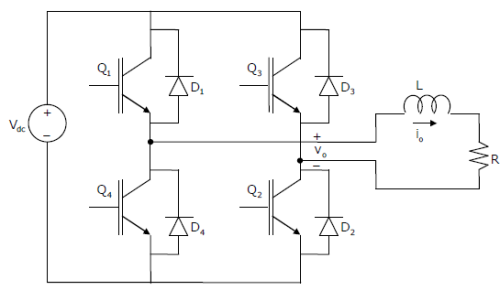In the interval when vo < 0 and io > 0 the pair of devices which conducts the load current is

Solution:

We consider the following two cases :
Case I : When Q Q1 2 , ON
In this case the +ve terminal of V0 will be at higher voltage. i.e. V0 > 0 and so
i0 > 0 (i.e., it will be +ve). Now, when the Q1, Q2 goes to OFF condition we
consider the second case.
Case II : When Q3, Q4 ON and Q, Q2 OFF :
In this condition, -ve terminal of applied voltage V0 will be at higher potential
i.e., V0 < 0 and since, inductor opposes the change in current so, although the
polarity of voltage V0 is inversed, current remains same in inductor i.e. I0 > 0.

This is the condition when conduction have been asked.

In this condition (V0 > 0, I0 > 0) since, IGBT’s can’t conduct reverse currents therefore current will flow through D D3 4 , until ID becomes negative. Thus, D3 and D4 conducts.

QUESTION: 65

The Voltage Source Inverter (VSI) shown in the figure below is switched to provide a 50Hz, square wave ac output voltage vo across an RL load. Reference polarity of vo and reference direction of the output current io are indicated in the figure. It is given that R = 3 ohms, L = 9.55mH.Appropriate transition i.e., Zero Voltage Switching (ZVS) / Zero Current Switching (ZCS) of the IGBTs during turn-on / turn-off is

Solution: